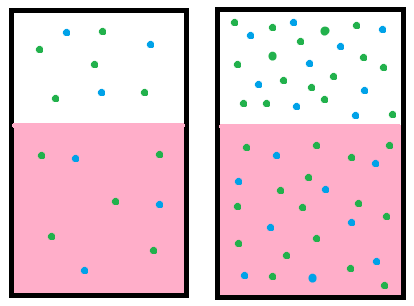# 21.8B: Henry’s Law

Henry’s law states that the amount of a gas that dissolves in a liquid is directly proportional to the partial pressure of that gas.

Learning Objectives

• Explain the way in which Henry’s law relates to gas exchange in the respiratory system

## Key Points

• At a constant temperature, the amount of a given gas that dissolves in a given type and volume of liquid is directly proportional to the partial pressure of that gas in equilibrium with that liquid.
• Gasses with a higher solubility will have more dissolved molecules than gasses with a lower solubility if they have the same partial pressure.
• Henry’s law explains how gasses dissolve across the alveoli – capillary barrier.
• Henry’s law predicts how gasses behave during gas exchange based on
the partial pressure gradients and solubility of oxygen and carbon
dioxide.

## Key Terms

• Henry’s law: At a constant temperature, the amount of a given gas that dissolves in a given type and volume of liquid is directly proportional to the partial pressure of that gas in equilibrium with that liquid.
• partial pressure gradient: The difference between the partial pressures (and thus concentration) of gasses between gaseous and dissolved forms.

## EXAMPLES

An everyday example of Henry’s law is given by carbonated soft drinks. Before the bottle or can is opened, the gas above the drink is almost pure carbon dioxide at a pressure slightly higher than atmospheric pressure. The drink itself contains dissolved carbon dioxide. When the bottle or can is opened, some of this gas escapes, giving the characteristic hiss (or pop in the case of a sparkling wine bottle). Because the pressure above the liquid is now lower, some of the dissolved carbon dioxide comes out of solution as bubbles. If a glass of the drink is left in the open, the concentration of carbon dioxide in solution will come into equilibrium with the carbon dioxide in the air, and the drink will go flat.

Henry’s law states that at a constant temperature, the amount of a gas that dissolves in a liquid is directly proportional to the partial pressure of that gas in equilibrium with that liquid. It was formulated by William Henry in 1803.Henry’s law: Henry’s law states that when a gas is in contact with the surface of a liquid, the amount of the gas which will go into solution is proportional to the partial pressure of that gas.

The practical description for the law is that the solubility (i.e., equilibrium) of a gas in a liquid is directly proportional to the partial pressure of that gas. In addition, the partial pressure is able to predict the tendency to dissolve simply because the gasses with higher partial pressures have more molecules and will bounce into the solution they can dissolve into more often than gasses with lower partial pressures.

Henry’s law also applies to the solubility of other substances that aren’t gaseous, such as the equilibrium of organic pollutants in water being based on the relative concentration of that pollutant in the media its suspended in.

Henry’s law can be put into mathematical terms (at constant temperature):

p=kHcp=kHc

Where p is the partial pressure of the solute in the gas above the solution, c is the concentration of the solute, the solubility of the substance is k, and the Henry’s law constant (H), which depends on the solute, the solvent, and the
temperature.

The solubility captures the tendency of a substance to go towards equilibrium in a solution, which explains why gasses that have the same partial pressure may have different tendencies to dissolve.

## Henry’s Law in Respiration

The main application of Henry’s law in respiratory physiology is to predict how gasses will dissolve in the alveoli and bloodstream during gas exchange. The amount of oxygen that dissolves into the bloodstream is directly proportional to the partial pressure of oxygen in alveolar air.

The partial pressure of oxygen is greater in alveolar air than in deoxygenated blood, so oxygen has a high tendency to dissolve into deoxygenated blood. Conversely the opposite is true for carbon dioxide, which has a greater partial pressure in deoxygenated blood than in the alveolar air, so it will diffuse out of the solution and back into gaseous form.

Recall that the difference in partial pressures between the bloodstream and alveoli (the partial pressure gradient) are much smaller for carbon dioxide compared to oxygen. Carbon dioxide has much higher solubility in the plasma of blood than oxygen (roughly 22 times greater), so more carbon dioxide molecules are able to diffuse across the small pressure gradient of the capillary and alveoli.

Oxygen has a larger partial pressure gradient to diffuse into the bloodstream, so it’s lower solubility in blood doesn’t hinder it during gas exchange. Therefore, based on the properties of Henry’s law, both the partial pressure and solubility of the oxygen and carbon dioxide determine how they will behave during gas exchange.